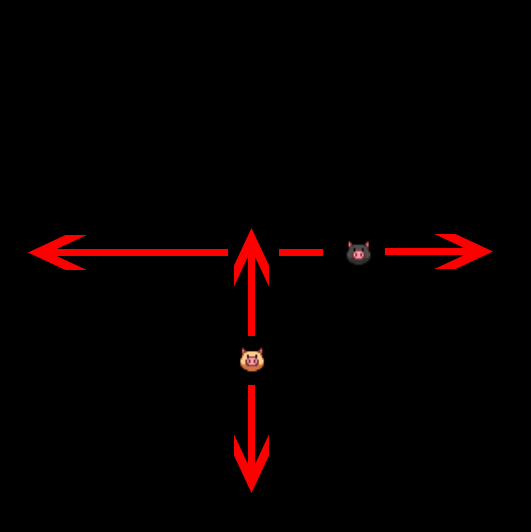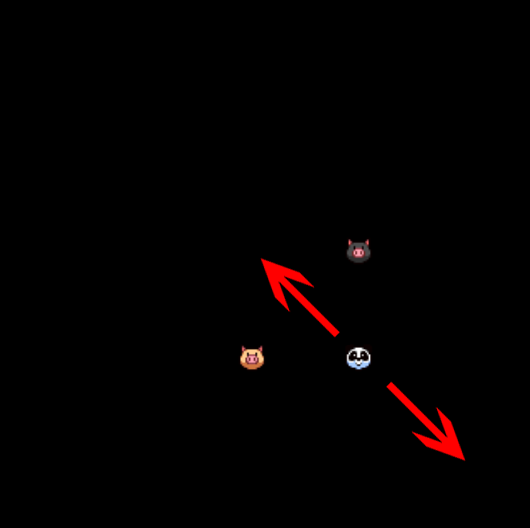# Enchant.js Guide

### 14 Plugin: Mixing Class Inheritance - mixing.enchant.js

You can reach the timeline plugin example here.

### 14.01 - A Word on mixing.enchant.js

enchant.js provides support for class inheritance, which you can read more about here.

On this note, the `mixing.enchant.js` plugin provides support for creating classes with multiple inheritance. The mixing method `enchant.Class.mixClasses()` takes in the parameters: `firstClass`, `secondClass`, `useOnlyOwnPropertiesForSecondclass`, and `initializeMethod`.

The `firstClass` and `secondClass` parameters are the two Classes you intend to mix.

The `useOnlyOwnProperties` is an optional boolean parameter that, if true, does not include properties given to it by the second class’s superclass. Not setting it to true can lead to strange behavior of a resulting class such as twicefold execution of methods provided by a shared superclass. By default, the flag is set to false.

`initializeMethod` is an optional method parameter that is used as the constructor for the resulting class.

### 14.02 - Mixing Example

In my simple example of the mixing plugin, I created two separate classes named A and B, with their code shown below.

Class A

``````
A = Class.create(Sprite, {
initialize: function(){
Sprite.call(this, 16, 16);
this.image = game.assets['icon0.png'];
this.x = stgWidth/2 - this.width;
this.y = stgHeight/2 - this.height;
this.moveVert = 2.0;
this.frame = 22;
},

onenterframe: function(){
this.y += this.moveVert;

if(this.y >= stgHeight - 20){
this.moveVert *= -1.0;
}
else if(this.y <= 20){
this.moveVert *= -1.0;
}
}
});
``````
Class B
``````
B = Class.create(Sprite, {
initialize: function(){
Sprite.call(this, 16, 16);
this.image = game.assets['icon0.png'];
this.x = stgWidth/2 - this.width;
this.y = stgHeight/2 - this.height;
this.moveHorz = 2.0;
this.frame = 23;
},

onenterframe: function(){
this.x += this.moveHorz;

if(this.x >= stgWidth - 20){
this.moveHorz *= -1.0;
}
else if(this.x <= 20){
this.moveHorz *= -1.0;
}
}
});
``````
In short, Class A moves left and right on the scene, and Class B moves up and down. This is pictured below in Figure 14.01.Figure 14.01: Class A and B

I then called the mixing method below like so:
``````
var C = enchant.Class.mixClasses(A, B, true);
``````
in order to create the Class C which inherits from the two classes. Also note that I’ve set `useOnlyOwnProperties` to true, and did not pass anything for `initializeMethod`.

Following this, I’ve created an instance of the Class C and added it to the scene. The result is a sprite that moves back and forth along both the X and Y axes in a diagonal fashion. This is pictured below in Figure 14.02.Figure 14.02: The Newly Created Class C